# DAV Class 6 Maths Chapter 4 Brain Teasers Solutions

The DAV Class 6 Maths Book Solutions Pdf and DAV Class 6 Maths Chapter 4 Brain Teasers Solutions of Ratio, Proportion and Unitary Method offer comprehensive answers to textbook questions.

## DAV Class 6 Maths Ch 4 Brain Teasers Solutions

Question 1A.

(a) The ratio of 1 cm to 1 mm is _______.
(i) 1 : 10
(ii) 10 : 1
(iii) 1 : 100
(iv) 100 : 1
Solution:
(ii) 10 : 1
1 cm : 1 mm
10 mm : 1 mm = 10 : 1
Hence, (ii) is the correct option.

(b) Fill in the box so that ___, 1, 0.5 are in continued proportion.
(i) 2
(ii) 0.4
(iii) 0.2
(iv) 4
Solution:
(i) 2
If x, 1, 0.5 are in continued proportion, then
$$\frac{x}{1}=\frac{1}{0.5}$$
∴ x = $$\frac{1}{0.5}=\frac{10}{5}$$ = 2
Hence, (i) is the correct option.(c) If 8 : 12 : : 26 : ___, then the number in the box is,
(i) 39
(ii) 12
(iii) 16
(iv) 18
Solution:
(i) 39
8 : 12 : : 26 : ___
8x = 12 × 26
x = $$\frac{12 \times 26}{8}$$ = 39
Hence, (i) is the correct option.

(d) The cost of six chocolates is ₹ 210, then the cost of four chocolates is _______
(i) ₹ 90
(ii) ₹ 120
(iii) ₹ 140
(iv) ₹ 100
Solution:
(iii) ₹ 140
Cost of 6 chocolates = ₹ 210
Cost of 1 chocolate = ₹ $$\frac{210}{6}$$
∴ Cost of 4 chocolates = ₹ $$\frac{210}{6}$$ × 4
= ₹ 140
Hence, (iii) is the correct option.

(e) The ratio of a dozen to a score is _______
(i) 5 : 3
(ii) 2 : 3
(iii) 3 : 2
(iv) 3 : 5
Solution:
(iv) 3 : 5
Dozen = 12, Score = 20
∴ Ratio of a dozen to a score = 12 : 20 = 3 : 5
Hence, (iv) is the correct option.(a) In the word ‘GEOMETRY’, find the ratio of vowels to the consonants.
Solution:
In word ‘GEOMETRY’, vowels are E, O, E and consonants G, M, T, R, Y.
∴ No. of vowels = 3 and
no. of consonants = 5
∴ Ratio of vowels to the consonants = 3 : 5

(b) bags of wheat weight 96 kg. How much will 20 similar bags weigh?
Solution:
Weight of 12 bags = 96 kg
Weight of 1 bag = $$\frac{96}{12}$$ kg = 8 kg
Weight of 20 bags = 8 × 20 = 160 kg

(c) Which is greater- 3 : 4 or 5 : 6?
Solution:
3 : 4 = $$\frac{3}{4}$$ = 0.75
and 5 : 6 = $$\frac{5}{6}$$ = 0.83 (approx.)
Clearly 0.83 > 0.75, so 5 : 6 is greater.

(d) Find the ratio of 125 ml to 5 litres.
Solution:
Ratio of 125 ml to 5 litres
= 125 ml : 5 litres
= 125 : 5000
= 1 : 40

(e) Find the number in the box if ___ : 6 : : 55 : 11.
Solution:
___ : 6 : : 55 : 11
11 × x = 6 × 55
x = $$\frac{6 \times 55}{11}$$
= 30
∴ x = 30Question 2.
Express the following ratios in the simplest form:
(a) 10 l to 0.25 l
(b) 2 hours to 45 seconds
(c) 13 weeks to 1 year
(d) 1 century to 1 decade
Solution:
(a) 10 l to 0.25 l = 10 : 0.25
= 10 × 100 : 0.25 × 100
= 1000 : 25
= 40 : 1
Hence, the lowest form = 40 : 1.

(b) 2 hours to 45 seconds
= 2 hrs : 45 sec
= 2 × 60 × 60 : 45
= 7200 : 45
= 160 : 1
Hence, the required ratio = 160 : 1.

(c) 13 weeks to 1 year = 13 weeks : 1 year
= 13 weeks : 52 weeks
= 13 : 52
= 1 : 4
Hence, the required ratio = 1 : 4.

(d) 1 century to 1 decade = 100 : 10
= 10 : 1
Hence, the required ratio = 10 : 1.Question 3.
Are the following in proportion?
(a) 16, 64, 18, 12
(b) 1.8, 4, 0.6, 5
Solution:
(a) 16, 64, 18, 72
If they are in proportion
∴ 16 : 64 : : 18 : 72
Product of extremes = 16 × 72 = 1152
Product of means = 64 × 18 = 1152
Hence they are in proportion.

(b) 1.8, 4, 0.6, 5
If they are in proportion
∴ 1.8 : 4 : : 0.6 : 5
Product of extremes = 1.8 × 5 = 9
Product of means = 4 × 0.6 = 2.4
9 ≠ 2.4
Hence they are not in proportion.

Question 4.
Fill in the boxes so that the numbers are in proportion:

(a) 24, 18, ___, 96
(b) 1000, 500, 200, ___
Solution:
(a) 24, 18, ___, 96
As the given numbers are in proportion
24 : 18 : :___ : 96
18 × ___= 24 × 96
___ =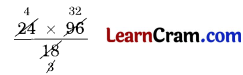= 128
Hence, ___ = 128

(b) 1000, 500, 200, ___
As the given number are in proportion
∴ 1000 : 500 : : 200 : ___
1000 × ___ = 500 × 200
___ =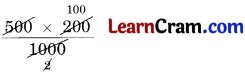= 100
Hence, ___ = 100Question 5.
Sahil weighs 36 kg. His brother weighs 2 kg more. Find the ratio of the weight of Sahil’s brother to Sahil.
Solution:
Weight of Sahil = 36 kg
Weight of his brother = 36 + 2 = 38 kg
∴ Ratio of Sahil’s brother weight to Sahil’s weight = 38 : 36
= 19 : 18
Hence, the required ratio = 19 : 18.

Question 6.
In an orchard, there are 150 trees. 65 of them are apple trees, 35 are pear trees and the rest are orange trees. Find the ratio of:
(а) Apple trees to pear trees
(b) Orange trees to apple trees
(c) Pear trees to total trees
Solution:
Total number of trees = 150
Number of apple trees = 65
Number of pear trees = 35
Number of orange trees = 150
∴ (65 + 35) = 50 trees

(a) Ratio of apple trees to pear trees = 65 : 35
= 13 : 7
Hence, the required ratio = 13 : 7.

(b) Ratio of orange trees to apple trees = 50 : 65
= 10 : 13
Hence, the required ratio = 10 : 13.

(c) Ratio of pear trees to total trees = 35 : 150
= 7 : 30
Hence, the required ratio = 7 : 30.Question 7.
Cost of one dozen oranges is ₹ 45:
(a) Find the cost of one score oranges.
(b) How many oranges can be purchased for ₹ 60?
Solution:
(a) 1 dozen = 12
and 1 score = 20
Cost of 12 oranges = ₹ 45
Cost of 1 orange = ₹ $$\frac{45}{12}$$
Cost of 20 oranges = ₹ $$\frac{45 \times 20}{12}$$
= ₹ 75
Hence, the required cost = ₹ 75

(b) ₹ 45 is the cost of 12 oranges 12
₹ 1 is the cost of $$\frac{12}{45$$ oranges
₹ 60 is the cost of $$\frac{12}{45$$ × 60 oranges = 16 oranges
Hence, the required number of oranges = 16.

Question 8.
Cost of 5 pizzas is ₹ 725 and 6 pastries is ₹ 210. If I want to purchase 3 pizzas and 12 pastries, how much should I pay?
Solution:
Cost of 5 pizzas = ₹ 725
Cost of 1 pizza = ₹ $$\frac{725}{5}$$
Cost of 3 pizzas = ₹ $$\frac{725}{5}$$ × 3 = ₹ 435
Cost of 6 pastries = ₹ 210
Cost of 1 pastry = ₹ $$\frac{210}{6}$$
Cost of 12 pastries = $$\frac{210}{6}$$ × 12 = 420
∴ Cost of 3 pizzas and 12 pastries = ₹ 435 + ₹ 420 = ₹ 855
Hence, the required cost = ₹ 855.Question 9.
What is the ratio of the
(a) number of even numbers to odd numbers from the set of natural numbers from 30 to 50?
(b) prime numbers and composite numbers from the set of natural numbers from 1 to 20?
Solution:
(a) Even numbers from 30 to 50 are 30, 32, 34, 36, 38, 40, 42, 44, 46, 48, 50
∴ Number of even numbers = 11
Odd numbers from 30 to 50 are 31, 33, 35, 37, 39, 41, 43, 45, 47, 49
∴ Number of odd numbers = 10
∴ Required ratio = 11 : 10.

(b) Composite numbers = 4, 6, 8, 9, 10, 12, 14, 15, 16, 18, 20
Number of composite number = 11
Prime number from 1 to 20 are 2, 3, 5, 7, 11, 13, 17, 19
Number of prime numbers = 8
∴ Required ratio = 8 : 11.

Question 10.
The sides of two squares are in the ratio 5 : 7. Find the ratio of their areas.
Solution:
Side of first square = 5x
Side of second square = 7x
Area of first square = (side)2
= (5x)2
= 25x2
Area of second square = (side)2
= (7x)2
= 49x2
∴ Ratio of areas of two squares = 25x2 : 49x2
= 25 : 49.

Question 11.
A distance of 500 km between two cities Raipur and Rajmundri is represented on the map by 5 cm. What is the actual distance between the cities if they are 8 cm apart on the map?
Solution:
Actual distance which is represented on map by 5 cm = 500 km
Actual distance which is represented on map by 1 cm = $$\frac{500}{5}$$ km
Actual distance which is represented on map by 8 cm
= $$\frac{500}{5}$$ km × 8
= 800 km### DAV Class 6 Maths Chapter 4 HOTS

Question 1.
The ratio of the length of a vertical pole and its shadow on the ground is 7 : 2. Find the length of the pole if the length of the shadow is 2.4 m.
Solution:
Length of vertical pole = 7x
Length of shadow of pole = 2x
∴ $$\frac{7 x}{2 x}=\frac{\text { length of pole }}{\text { length of shadow }}$$
⇒ $$\frac{7 x}{2 x}=\frac{\text { length of pole }}{2.4 \mathrm{~m}}$$
∴ Length of pole = $$\frac{7 x}{2 x}$$ × 2.4 m = 8.4 mQuestion 1.
Express the following ratios in the simplest form:
(a) 4 days to 240 hours
(b) 1 year to 52 weeks
(c) 8 hours to 120 minutes
(d) 4 dozen to 1 score
Solution:
(a) 4 days to 240 hours = 4 × 24 : 240
= 96 : 240
= 2 : 5
Hence, the required ratio is 2 : 5.

(b) 1 year to 52 weeks = 1 × 52 weeks : 52 weeks
= 52 : 52
= 1 : 1
Hence, the required ratio is 1 : 1.

(c) 8 hours to 120 minutes = 8 × 60 min : 120 min
= 480 : 120
= 4 : 1
Hence the required ratio is 4 : 1.

(d) 4 dozen to 1 score = 4 × 12 : 1 × 60
= 48 : 60
= 4 : 5
Hence, the required ratio is 4 : 5.Question 2.
There are 1600 students in a school out of which 1200 are boys. Find:
(а) ratio of boys to girls
(b) ratio of boys to total students
(c) ratio of girls to total students
(d) ratio of difference of boys and girls to the total students.
Solution:
Total number of students = 1600
Number of boys = 1200
∴ Number of girls = 1600 – 1200 = 400
Difference of boys and girls = 1200 – 400 = 800

(а) ratio of boys to girls = 1200 : 400
= 3 : 1
Hence, the required ratio is 3 : 1.

(b) ratio of boys to total students = 1200 : 1600
= 3 : 4
Hence, the required ratio is 3 : 4.

(c) Ratio of girls to total students = 400 : 1600
= 1 : 4
Hence, the required ratio is 1 : 4.

(d) ratio of difference of boys and girls to total students = 800 : 1600
= 1 : 2
Hence, the required ratio is 1 : 2.Question 3.
Fill in the blanks:
(а) 25 : 35 : : 35 : ___
(b) ___ : 8 : : 5 : 10
(c) 12 : ___ : : 8 : 6
(d) 12 : 16 : : ___ : 8
Solution:
(a) 25 : 35 : : 35 : ___
⇒ 25 × ___ = 35 × 35
⇒ ___ = $$\frac{35 \times 35}{25}$$
∴ ___ = 49

(b) ___ : 8 : : 5 : 10
⇒ ___ × 10 = 8 × 5
⇒ ___ =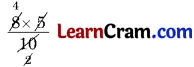∴ ___ = 4

(c) 12 : ___ : : 8 : 6
12 × 6 = ___ × 8
⇒ ___ = $$\frac{12 \times 6}{8}$$
∴ ___ = 9

(d) 12 : 16 : : ___ : 8
⇒ 16 × ___= 12 × 8
⇒ ___ = $$\frac{12 \times 8}{16}$$
∴ ___ = 6Question 4.
In the word MISSISSIPPI, find:
(a) ratio of Is to total number of alphabet.
(b) ratio of Ss to total number of alphabet.
(c) ratio of Is to Ss.
(d) ratio of Ps to total number of alphabet.
Solution:
In MISSISSIPPI
Total number of alphabet =11
Number of Is = 4
Number of Ss = 4
Number of P = 2

(a) ratio of Is to total number of alphabet = 4 : 11

(b) ratio of Ss to total number of alphabet = 4 : 11

(c) ratio of Is to Ss = 4 : 4 = 1 : 1

(d) ratio of Ps to total number of alphabet = 2 : 11

Question 5.
Are the following in proportion?
(a) 20, 18, 40, 36
(b) 15, 20, 35, 40
Solution:
(a) If 20, 18, 40, 36 are in proportion
∴ 20 : 18 : : 40 : 36
Product of extremes = 20 × 36 = 720
Product of means = 18 × 40 = 720
Hence, they are in proportion.

(b) If 15, 20, 35, 40 are in proportion
∴ 15 : 20 : : 35 : 40
Product of extremes = 15 × 40 = 600
Product of means = 20 × 35 = 700
600 ≠ 700
Hence, the given numbers are not in proportion.Question 6.
Fill in the blanks:

(а) A proportion has _______ terms.
Solution:
two

(b) : : is the symbol for _______.
Solution:
proportion

(c) The product of extremes must be equal to the product of the _______ if the numbers are in proportion.
Solution:
means

(d) Equality of two ratio is called _______.
Solution:
proportion

(e) The second and third terms of a proportion are called _______.
Solution:
means

(f) The first and fourth terms of a proportion are called _______.
Solution:
extremes

(g) The ratio is always expressed in its _______ form.
Solution:
simplest

(h) Comparisons of two quantities of same units is called _______.
Solution:
ratioQuestion 7.
Cost of one dozen oranges is ₹ 21:
(a) Find the cost of one score oranges.
(b) How many oranges can be purchased for ₹ 19.25?
Solution:
(a) 1 dozen = 12
and 1 score = 60
Cost of 12 oranges = 21
Cost of 1 orange = ₹
Cost of 60 oranges = ₹ $$\frac{21 \times 60}{12}$$
= ₹ 21 × 5 = ₹ 105
Hence, the required cost = ₹ 105

(b) ₹ 21 is the cost of 12 oranges
₹ 1 is the cost of $$\frac{12}{21}$$ oranges
₹ 19.25 is the cost of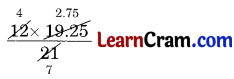= 4 × 2.75
= 11.00 oranges
Hence, the required number of oranges = 11.Question 8.
Cost of 5 pizzas is ₹ 725 and 6 pastries is ₹ 54. If I want to purchase 3 pizzas and 12 pastries, how much should I pay?
Solution:
Cost of 5 pizzas = ₹ 725
Cost of 1 pizza = ₹ $$\frac{725}{5}$$
Cost of 3 pizzas =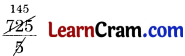× 3
= ₹ 145 × 3
= ₹ 435
Cost of 6 pastries = ₹ 54
Cost of 1 pastry = ₹ $$\frac{54}{6}$$
Cost of 12 pastries = $$\frac{54}{6}$$ × 12
= ₹ 54 × 2 = ₹ 108
Cost of 3 pizzas and 12 pastries = ₹ 435 + ₹ 108 = ₹ 543
Hence, the required cost = ₹ 543.